# E-PolyLearning

 1. Choose the figure which is different from the rest.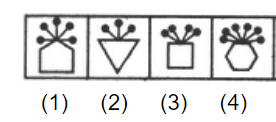a. 1 b. 2 c. 3 d. 4

 2. Choose the figure which is different from the rest.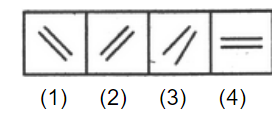a. 1 b. 2 c. 3 d. 4
 3. Choose the figure which is different from the rest.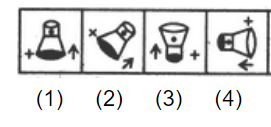a. 1 b. 2 c. 3 d. 4
 4. Choose the figure which is different from the rest.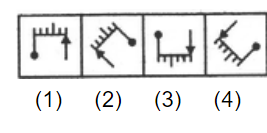a. 1 b. 2 c. 3 d. 4
 5. Choose the figure which is different from the rest.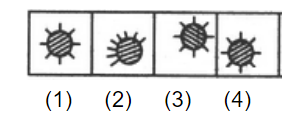a. 1 b. 2 c. 3 d. 4